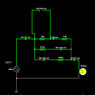# Why this Simple Watt Meter for a 220 Volt Circuit works?

• henriquevpp

#### henriquevpp

TL;DR Summary
Help with a wattmeter for 220V circuits that have only resistors.
Hello guys!

I'm just a begginer student. I found this circuit of a wattmeter that works only with resistors. Can someone explain to me how the 33k resistor give a voltage of 1 mV to 1 Watt? I did not understand why these resistor values has been chosen and why the it measures only in the 33k resistor.

I made a version (only changing one resistor value) at Fasltad: http://tinyurl.com/y7uqu8n4 (the url was too big).

Can someone help me? Thank you!

#### Attachments

•1589328541380.png
5.2 KB · Views: 145

The two 470m resistors are 0.47 ohm current shunts in parallel, so they drop a voltage proportional to the current flowing in the light globe load. Vshunt = I * 0.235

That small AC voltage is attenuated by the resistive divider, 33K and 7k5 to be measured.
The supply voltage is reasonably fixed so the small AC voltage across the 33k is proportional to the load power.

•dlgoff, Merlin3189 and henriquevpp
1 watt will have a load current of 1 / 220 = 4.545 milliamp.
Since then V * I = 220 V * 4.545 mA = 1 watt. (edited units on this line).
The two 470m current shunts make 0.235 ohm.
0.235 ohm * 4.545 mA = 1.068 mV per watt.
1.068 * 33k/(33k+7k5) = 0.870 mV per watt. Which is wrong.

If we assume the AC voltmeter has a very high impedance.
The divider needs a ratio of 1 / 1.068 = 0.936
What value should be used in place of 7k5 ?
33k / ( 33k + x ) = 0.936
x = ( 33k / 0.936 ) - 33k = 2.25k, not 7k5

Last edited:
•dlgoff, Tom.G and henriquevpp
Odd that the current is shown as 524 mA, which from a 220 V supply sounds like around 115 W not 100.

And now Baluncore has done the rest

•henriquevpp and Baluncore
And then again such a "wattmeter" assumes that the voltage and current are in phase. Creating a true RMS wattmeter is tricky (but possible, a colleague and I dreamed up such a circuit 15 years ago).